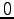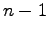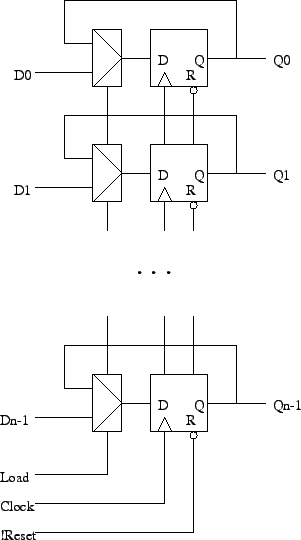Registers

Tom Kelliher, CS 240

Apr. 26, 2010

Announcements

Homework due today!

From Last Time

VHDL for sequential circuits.

Outline

1. Definitions.

2. Parallel registers.

3. VHDL for registers.

Shift registers.

Definitions

1. Register: A set of flip-flops which have common clock and control signals and load related bits -- a whole word, data on a bus.

Examples: register file, MDR, MAR.

2. Counter: A register which passes through a pre-determined set of states. Usually they count by one fromto.

Example: program counter.

Parallel Registers

Use a mux approach:Gating the clock will save the muxes, but clock gating is usually a bad idea -- clock skew problems.

1. Really bad: `clockToFF = load and clock`

2. Just as bad: `clockToFF = !load or clock`

Show waveforms and show what skew looks like and why we care.

VHDL for Registers

Note: The handling of the load signal could be used to implement a synchronous reset.

```-- VHDL for 32 bit parallel load register with asynchronous low
-- reset.

library ieee;
use ieee.std_logic_1164.all;

entity parallel_register is

port (
clock, load, reset_n : in  std_logic;
d                    : in  std_logic_vector (31 downto 0);
q                    : out std_logic_vector (31 downto 0));

end parallel_register;

architecture dataflow of parallel_register is

signal state : std_logic_vector (31 downto 0);

begin  -- dataflow

q <= state;                           -- Output process.

state_reg : process (clock, reset_n)
begin  -- process state
if (reset_n = '0') then             -- asynchronous reset (active low)
state <= X"00000000";
elsif (clock'event and clock = '1') then  -- rising clock edge
state <= d;
else
state <= state;
end if;
end if;
end process state_reg;

end dataflow;
```

Thomas P. Kelliher 2010-04-26
Tom Kelliher